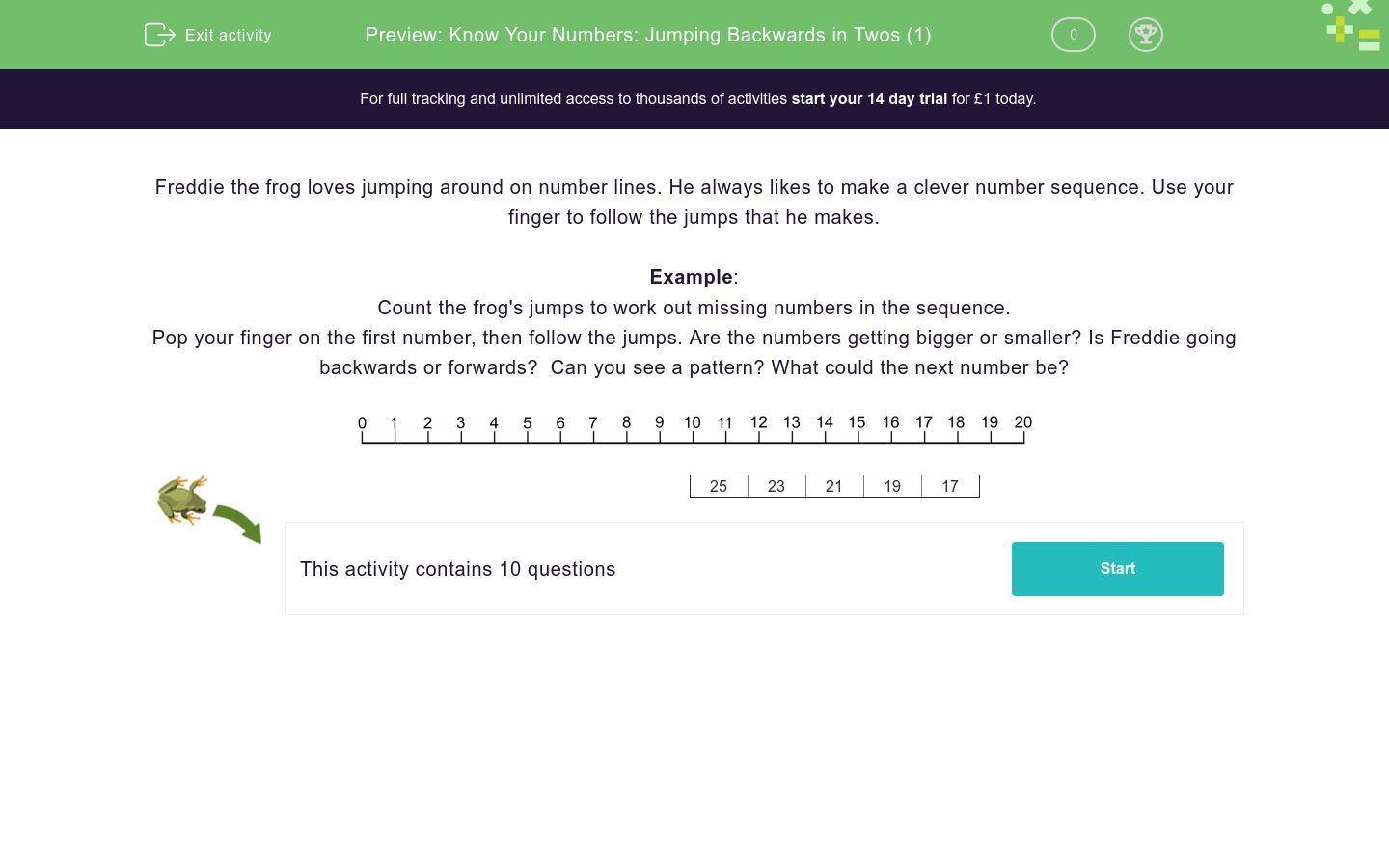# Know Your Numbers: Jumping Backwards in Twos (1)

In this worksheet, students count backwards in twos and work out the missing gaps in a number sequences.Key stage:  KS 1

Curriculum topic:   Number: Number and Place Value

Curriculum subtopic:   Count in Steps (2, 3, 5 and 10)

Difficulty level:### QUESTION 1 of 10

Freddie the frog loves jumping around on number lines. He always likes to make a clever number sequence. Use your finger to follow the jumps that he makes.

Example:

Count the frog's jumps to work out missing numbers in the sequence.

Pop your finger on the first number, then follow the jumps. Are the numbers getting bigger or smaller? Is Freddie going backwards or forwards?  Can you see a pattern? What could the next number be?25 23 21 19 17

Count the frog's jumps to work out missing numbers in the sequence.21 19 17 15 a b
a=13 and b=11

a=11 and b=18

a=21 and b=17

Count the frog's jumps to work out missing numbers in the sequence.15 13 11 9 a b
a=5 and b=7

a=7 and b=5

a=7 and b=8

Count the frog's jumps to work out missing numbers in the sequence.14 12 10 8 a b
a=4 and b=5

a=8 and b=7

a=6 and b=4

Count the frog's jumps to work out missing numbers in the sequence.13 11 9 7 a b
a=5 and b=3

a=5 and b=4

a=3 and b=4

Count the frog's jumps to work out missing numbers in the sequence.11 9 7 5 a b
a=3 and b=4

a=3 and b=1

a=1 and b=2

Count the frog's jumps to work out missing numbers in the sequence.12 10 8 6 a b
a=5 and b=3

a=4 and b=3

a=4 and b=2

Count the frog's jumps to work out missing numbers in the sequence.18 16 14 12 a b
a=10 and b=11

a=8 and b=10

a=10 and b=8

Count the frog's jumps to work out missing numbers in the sequence.20 18 16 14 a b
a=12 and b= 11

a=12 and b= 10

a=11 and b= 9

Count the frog's jumps to work out missing numbers in the sequence.22 20 18 16 a b
a=21 and b=17

a=14 and b=12

a=20 and b=19

Count the frog's jumps to work out missing numbers in the sequence.24 22 20 18 a b
a=16 and b=14

a=12 and b=10

a=11 and b=13

• Question 1

Count the frog's jumps to work out missing numbers in the sequence.21 19 17 15 a b
a=13 and b=11
EDDIE SAYS
21, 19, 17, 15, 13, 11 is the sequence. Freddie is jumping back 2 places each time.
• Question 2

Count the frog's jumps to work out missing numbers in the sequence.15 13 11 9 a b
a=7 and b=5
EDDIE SAYS
15, 13, 11, 9, 7, 5 is the sequence. Freddie is jumping back 2 places each time.
• Question 3

Count the frog's jumps to work out missing numbers in the sequence.14 12 10 8 a b
a=6 and b=4
EDDIE SAYS
14, 12, 10, 8, 6, 4 is the sequence. Freddie is jumping back 2 places each time.
• Question 4

Count the frog's jumps to work out missing numbers in the sequence.13 11 9 7 a b
a=5 and b=3
EDDIE SAYS
13, 11, 9, 7, 5, 3 is the sequence. Freddie is jumping back 2 places each time.
• Question 5

Count the frog's jumps to work out missing numbers in the sequence.11 9 7 5 a b
a=3 and b=1
EDDIE SAYS
11, 9, 7, 5, 3, 1 is the sequence. Freddie is jumping back 2 places each time.
• Question 6

Count the frog's jumps to work out missing numbers in the sequence.12 10 8 6 a b
a=4 and b=2
EDDIE SAYS
12, 10, 8, 7, 6, 4 is the sequence. Freddie is jumping back 2 places each time.
• Question 7

Count the frog's jumps to work out missing numbers in the sequence.18 16 14 12 a b
a=10 and b=8
EDDIE SAYS
18, 16, 14, 12, 10, 8 is the sequence. Freddie is jumping back 2 places each time.
• Question 8

Count the frog's jumps to work out missing numbers in the sequence.20 18 16 14 a b
a=12 and b= 10
EDDIE SAYS
20, 18, 16, 14, 12, 10 is the sequence. Freddie is jumping back 2 places each time.
• Question 9

Count the frog's jumps to work out missing numbers in the sequence.22 20 18 16 a b
a=14 and b=12
EDDIE SAYS
22, 20, 18, 16, 14, 12 is the sequence. Freddie is jumping back 2 places each time.
• Question 10

Count the frog's jumps to work out missing numbers in the sequence.24 22 20 18 a b
a=16 and b=14
EDDIE SAYS
24, 22, 20, 18, 16, 14 is the sequence. Freddie is jumping back 2 places each time.
---- OR ----

Sign up for a £1 trial so you can track and measure your child's progress on this activity.

### What is EdPlace?

We're your National Curriculum aligned online education content provider helping each child succeed in English, maths and science from year 1 to GCSE. With an EdPlace account you’ll be able to track and measure progress, helping each child achieve their best. We build confidence and attainment by personalising each child’s learning at a level that suits them.

Get started